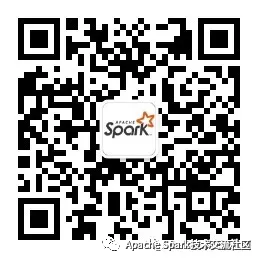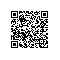# Apache Spark 3.0 中的向量化 IO

R 是数据科学中最流行的计算机语言之一，专门用于统计分析和一些扩展，如用于数据处理和机器学习任务的 RStudio addins 和其他 R 包。此外，它使数据科学家能够轻松地可视化他们的数据集。

### Spark 和 R 交互

SparkR 不仅支持丰富的 ML 和类似 SQL 的 API 集合，而且还支持用于直接与 R 代码进行交互的一组 API。例如，Spark DataFrame 和 R DataFrame 之间的无缝转换以及在 Spark DataFrame 上以分布式的方式执行 R 内置函数。

// Scala API
// ~1 second
sql("SELECT id FROM range(2000000000)").filter("id > 10").count()

# R API
# ~1 second
count(filter(sql("SELECT * FROM range(2000000000)"), "id > 10"))

// Scala API
val ds = (1L to 100000L).toDS
// ~1 second
ds.mapPartitions(iter => iter.filter(_ < 50000)).count()

# R API
df <- createDataFrame(lapply(seq(100000), function (e) list(value=e)))
# ~15 seconds - 15 times slower
count(dapply(
df, function(x) as.data.frame(x[x\$value < 50000,]), schema(df)))

// Scala API
// ~0.2 seconds
val df = sql("SELECT * FROM range(1000000)").collect()

# R API
# ~8 seconds - 40 times slower
df <- collect(sql("SELECT * FROM range(1000000)"))

createDataFrame()
collect()
dapply()
dapplyCollect()
gapply()
gapplyCollect()

### 原始实现（Native implementation）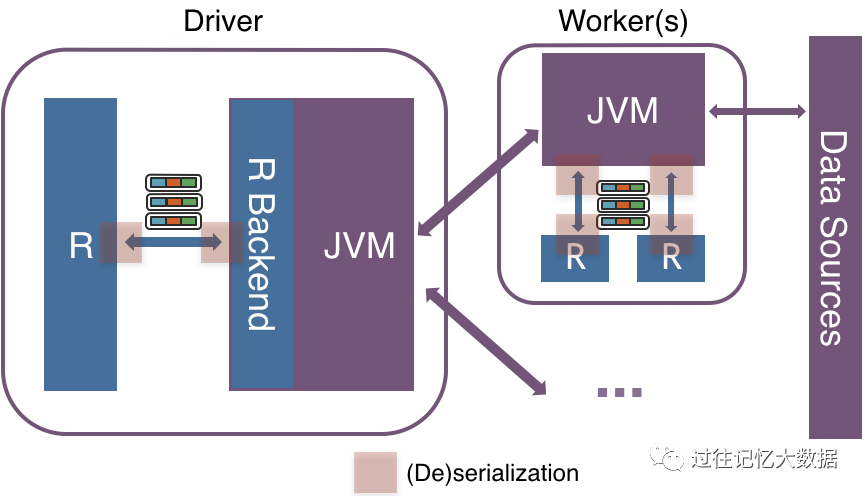### 向量化执行（Vectorized implementation）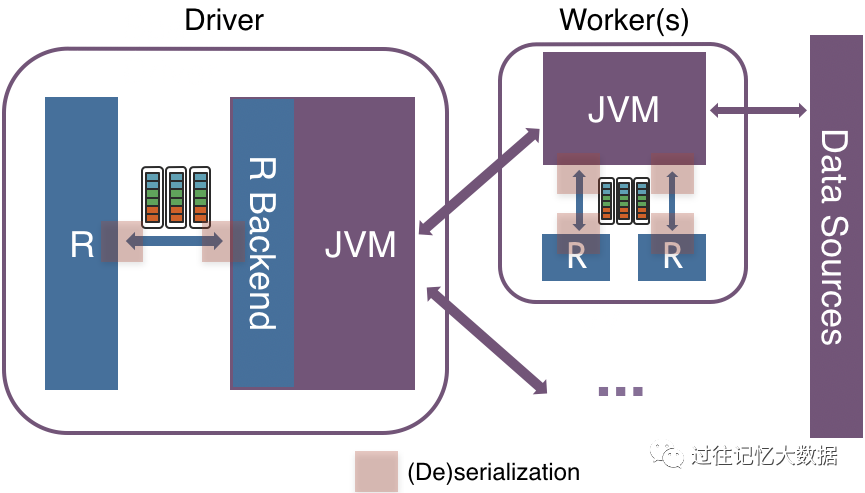### 基准测试结果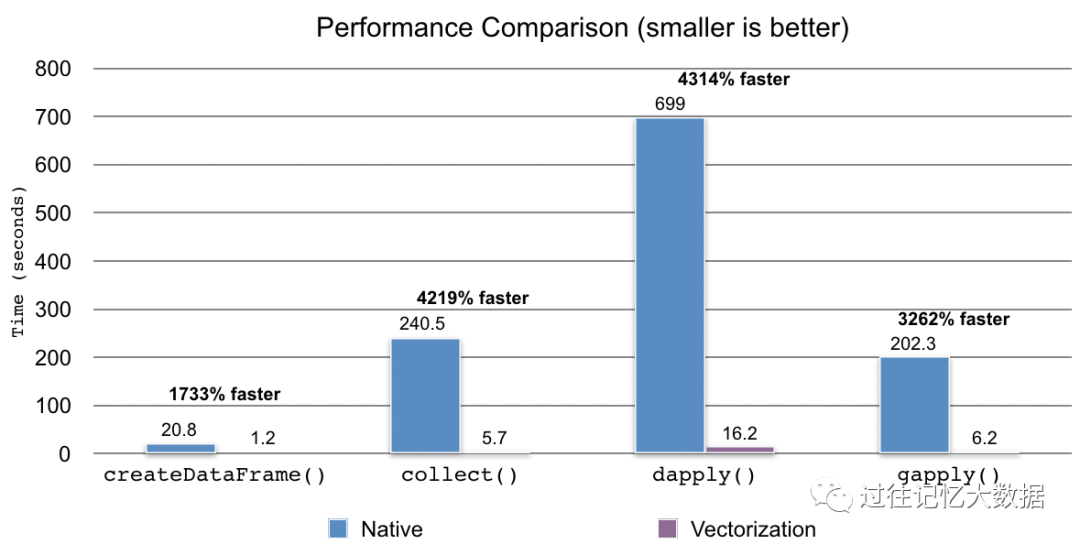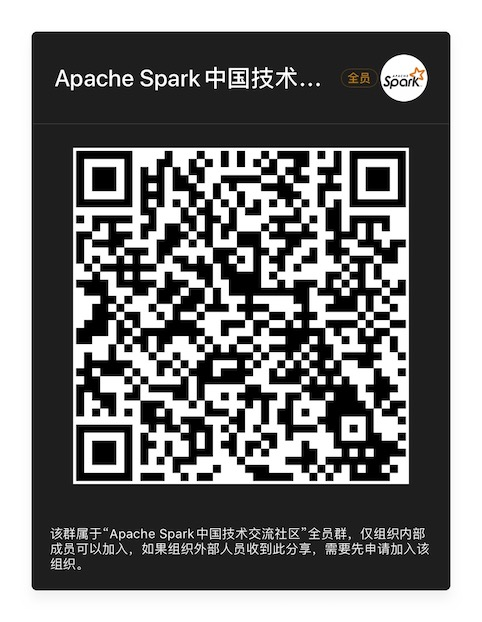Apache Spark技术交流社区公众号，微信扫一扫关注